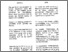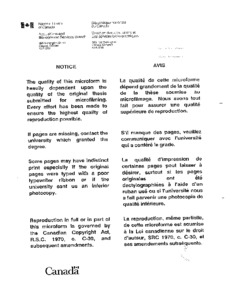Title:

# Hot deformation behaviour of SiCp/Al composites and their matrices and an Al-Mg-Si alloy

Sakaris, Peter (1993) Hot deformation behaviour of SiCp/Al composites and their matrices and an Al-Mg-Si alloy. Masters thesis, Concordia University.Preview
Text (application/pdf)
MM84699.pdf
3MB

## Abstract

Isothermal hot torsion tests were performed on two metal matrix composites (15v/o SiC$\sb{\rm p}$/A356 Al and 15v/o SiC$\sb{\rm p}$/6061 Al), a casting aluminum-silicon matrix alloy (A356) and an aluminum-magnesium-silicon alloy (6201). The composites and matrix alloy were tested over the range 300 to 540$\sp\circ$C and 0.1 to 5.0 s$\sp{-1}$. Flow stress ($\sigma$) and strain-to-failure ($\varepsilon\sb{\rm f}$) data were determined and compared with published results. The flow stresses decrease monotonically with increasing deformation temperature (T), following the same relationship as the published data. At very high temperatures, $\sigma$ is similar for all materials but as T falls to 300$\sp\circ$C, the A356 rises more rapidly than 6061. Flow stresses for the composites rise more rapidly than the matrix alloys. The ductility of all materials increases as T increases although it did not rise nearly as much for A356 as for 6061. The decreased reduction in $\varepsilon\sb{\rm f}$ in the A356 materials compared to the 6061 materials indicates that the SiC particles have much less effect as additional crack initiators in A356. The plot of log $\.\varepsilon$ versus log sinh $\alpha\sigma$ were approximately linear over the narrow range of strain rates tested. Testing of 6201 Al was conducted at 200 to 500$\sp\circ$C for overaged specimens and at 300 to 600$\sp\circ$C for the solutioned ones at rates of 0.1 to 4.0 s$\sp{-1}$. The activation energy for the precipitated alloy is about 192 kJ/mol which is higher than that of pure aluminum. The alloy is somewhat stronger than aluminum because of the particles at low temperatures and the increased level of solute at high ones. The solutional specimens had high peak stresses and considerable work softening at 300$\sp\circ$C due to dynamic formation and coalescence of precipitates. The Q$\sb{\rm HW}$ was higher but poorly defined because of variations in precipitation during heating. The ductility increased as T rose and $\.\varepsilon$ decreased and was higher for precipitated than for solutioned specimens. (Abstract shortened by UMI.)

Divisions: Concordia University > Gina Cody School of Engineering and Computer Science > Mechanical and Industrial Engineering Thesis (Masters) Sakaris, Peter xv, 124 leaves : ill. ; 29 cm. Concordia University M.A. Sc. Dept. of Mechanical Engineering 1993 McQueen, Hugh J. 43 Concordia University Library 27 Aug 2009 17:09 18 Jan 2018 17:12 http://clues.concordia.ca/search/c?SEARC...
All items in Spectrum are protected by copyright, with all rights reserved. The use of items is governed by Spectrum's terms of access.

Repository Staff Only: item control pageResearch related to the current document (at the CORE website)
Back to top